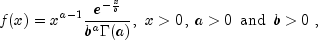JMSLTM Numerical Library 7.2.0
com.imsl.stat

• All Implemented Interfaces:
Distribution, ProbabilityDistribution, Serializable

```public class GammaDistribution
extends Object
implements ProbabilityDistribution, Serializable```
Evaluates a gamma probability density for a given set of data.

`GammaDistribution` evaluates the gamma density of a given set of data, `xData`. If parameters are not supplied, the `Eval` method fits the gamma probability density function to the data by first calculating the shape and scale parameters using an MLE technique for a best fit. The gamma probability density function is defined as:where a and b are the scale and shape parameters.

The `DataMining` package class `NaiveBayesClassifier` uses `GammaDistribution` as a method to train continuous data.

Example, Serialized Form
• ### Constructor Summary

Constructors
Constructor and Description
`GammaDistribution()`
• ### Method Summary

Methods
Modifier and Type Method and Description
`double[]` `eval(double[] xData)`
Fits a gamma probability distribution to `xData` and returns the probability density at each value.
`double[]` ```eval(double[] xData, Object[] parameters)```
Evaluates a gamma probability distribution with a given set of parameters at each point in `xData` and returns the probability density at each value.
`double` ```eval(double xData, Object[] parameters)```
Evaluates a gamma probability density at a given point `xData`.
`Object[]` `getParameters()`
Returns the current parameters of the gamma probability density function.
`double` `getScaleParameter()`
Returns the maximum-likelihood estimate found for the gamma scale parameter.
`double` `getShapeParameter()`
Returns the maximum-likelihood estimate found for the gamma shape parameter.
• ### Methods inherited from class java.lang.Object

`clone, equals, finalize, getClass, hashCode, notify, notifyAll, toString, wait, wait, wait`
• ### Constructor Detail

`public GammaDistribution()`
• ### Method Detail

• #### eval

`public double[] eval(double[] xData)`
Fits a gamma probability distribution to `xData` and returns the probability density at each value.
Specified by:
`eval` in interface `Distribution`
Parameters:
`xData` - a `double` array representing the points at which the gamma probability distribution function is to be evaluated
Returns:
a `double` array representing the gamma probability density at each value of `xData`
• #### eval

```public double[] eval(double[] xData,
Object[] parameters)```
Evaluates a gamma probability distribution with a given set of parameters at each point in `xData` and returns the probability density at each value.
Specified by:
`eval` in interface `ProbabilityDistribution`
Parameters:
`xData` - a `double` array representing the points at which the gamma probability distribution function is to be evaluated
`parameters` - an `Object` array representing the parameters used to evaluate the gamma distribution, see method `getParameters`
Returns:
a `double` array representing the gamma probability density at each value of `xData`
• #### eval

```public double eval(double xData,
Object[] parameters)```
Evaluates a gamma probability density at a given point `xData`.
Specified by:
`eval` in interface `ProbabilityDistribution`
Parameters:
`xData` - a `double` representing the point at which the gamma probability distribution function is to be evaluated
`parameters` - an `Object` array representing the parameters used to evaluate the gamma distribution, see method `getParameters`
Returns:
a `double` representing the gamma probability density at `xData`
• #### getParameters

`public Object[] getParameters()`
Returns the current parameters of the gamma probability density function.
Specified by:
`getParameters` in interface `ProbabilityDistribution`
Returns:
an `Object` array containing the parameters resulting from the last invocation of the (`Distribution`) `eval` method with the following signature, `double[] eval(double[] xData)`. This `Object` array can be used as input to the `eval` methods that require an `Object` array of distribution parameters as input.
• #### getScaleParameter

`public double getScaleParameter()`
Returns the maximum-likelihood estimate found for the gamma scale parameter.
Returns:
a `double` representing the maximum-likelihood estimate found for the gamma scale parameter
• #### getShapeParameter

`public double getShapeParameter()`
Returns the maximum-likelihood estimate found for the gamma shape parameter.
Returns:
a `double` representing the maximum-likelihood estimate found for the gamma shape parameter
JMSLTM Numerical Library 7.2.0